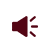• ISSN 1001-1455  CN 51-1148/O3
• EI、Scopus、CA、JST收录
• 力学类中文核心期刊
• 中国科技核心期刊、CSCD统计源期刊引用本文: 郑永辉, 魏继锋. 水介质初始参数设置对水下爆炸载荷的影响[J]. 爆炸与冲击, 2022, 42(5): 053202.ZHENG Yonghui, WEI Jifeng. Effect of initial parameter setting of water on load characteristics of underwater explosion[J]. Explosion And Shock Waves, 2022, 42(5): 053202. doi: 10.11883/bzycj-2021-0485
 Citation: ZHENG Yonghui, WEI Jifeng. Effect of initial parameter setting of water on load characteristics of underwater explosion[J]. Explosion And Shock Waves, 2022, 42(5): 053202.## 水介质初始参数设置对水下爆炸载荷的影响

##### doi: 10.11883/bzycj-2021-0485

###### 通讯作者: 魏继锋（1977－　），男，博士，副教授，weijifeng@bit.edu.cn
• 中图分类号: O389；TJ02

## Effect of initial parameter setting of water on load characteristics of underwater explosion

• 摘要: 针对数值计算中水介质初始参数设置对水下爆炸载荷特性的影响开展了深入分析。基于参考状态参数确定了水介质状态方程形式；从热力学角度分析了常用的两种初始参数设置方式，提出了一种按等温假设设置初始参数的方式，并对LS-DYNA中INITIAL_EOS_ALE关键字给出的参数设置结果进行了分析；采用LS-DYNA程序进行一维球形装药水下爆炸数值计算，分析了3种设置方式下爆炸载荷特性的差异，并与已有研究成果进行了对比。结果表明：当仅改变水介质内能项时，参数按等容过程变化，流场压力源于外界传热，与实际深水环境严重不符；INITIAL_EOS_ALE关键字给出的参数设置结果与仅改变水介质密度（等内能过程）接近，水温变化规律与真实环境不符；按等内能过程和等温过程设置初始参数时，水下爆炸载荷特性计算结果基本相同，与已有成果吻合；综合分析认为，按等温形式进行初始参数设置方式较优。研究成果可为水下爆炸尤其是深水爆炸数值仿真提供参考。
• 图  1  不同设置方式下的μeV和ΔT

Figure  1.  Values of μ, eV and ΔT in different setting modes

图  2  不同设置方式下的声速变化

Figure  2.  Acoustic velocity in different modes

图  3  H=5 km及R=55R0时3种方式下的冲击波压力-时间曲线

Figure  3.  Shock wave pressure-time curves in three modes when H=5 km and R=55R0

图  4  R=55R0时不同水深下3种方式所对应的冲击波载荷参数

Figure  4.  Values of shock wave load in three modes when R=55R0

图  5  H=5 km时3种方式下气泡的半径-时间曲线

Figure  5.  Bubble radius-time curves in three modes when H=5 km

图  6  Vanzant等的试验结果及线性拟合曲线

Figure  6.  Test results from Vanzant et al and linear fitting results

图  7  ΔPm在5 km水深处相对于在0 m处的变化幅度

Figure  7.  Changing amplitudes of ΔPm when depth changes from 0 m to 5 km

图  8  H=5 km时ΔPm相对于计算式(8)的误差

Figure  8.  Relative errors of ΔPm between simulation results and calculation formulas when H=5 km

图  9  气泡脉动参数相对于经验值的误差

Figure  9.  Relative errors of Rmax and T between simulation and empirical formula

表  1  水介质状态方程参数

Table  1.   EOS parameters of water

 C0/Pa C1/GPa C2/GPa C3/GPa C4 C5 C6 101325 2.2 9.54 14.57 0.28 0.28 0

表  2  $H=5\;{\rm {km}}$$R=55R_0$时3种方式下的$t_{\rm a}$$\Delta P_{\rm m}$$t_{\rm c}$Table  2.   Values of $t_{\rm a}$, $\Delta P_{\rm m}$and $t_{\rm c}$in three modes when $H=5\;{\rm {km}}$and $R=55R_0$方式 ta/ms ΔPm/MPa tc/ms Ⅰ 1.7745 12.080 0.2676 Ⅱ 1.6578 13.603 0.2587 Ⅲ 1.6518 13.692 0.2581

表  3  冲击波载荷在5 km水深处相对于0 m的变化幅度

Table  3.   Changing amplitudes of shock wave load when depth changes from 0 m to 5 km

 方式 变化幅度/% ΔPm I es Ⅰ −1.202 −74.304 −32.896 Ⅱ 11.255 −73.227 −21.877 Ⅲ 11.981 −73.171 −21.247

表  4  $H=5\;{\rm{km}}$时3种方式下对应的$R_{\max}$$T$Table  4.   Values of $R_{\max}$and $T$in three modes when $H=5\;{\rm{km}}$方式 Rmax/mm T/ms Ⅰ 178.722 1.7129 Ⅱ 180.618 1.7445 Ⅲ 180.731 1.7460
•点击查看大图
##### 计量
• 文章访问数:  138
• HTML全文浏览量:  61
• PDF下载量:  74
• 被引次数: 0
##### 出版历程
• 收稿日期:  2021-11-18
• 修回日期:  2021-12-25
• 网络出版日期:  2022-04-25
• 刊出日期:  2022-05-27

### 目录/下载:  全尺寸图片 幻灯片
• 分享
• 用微信扫码二维码

分享至好友和朋友圈